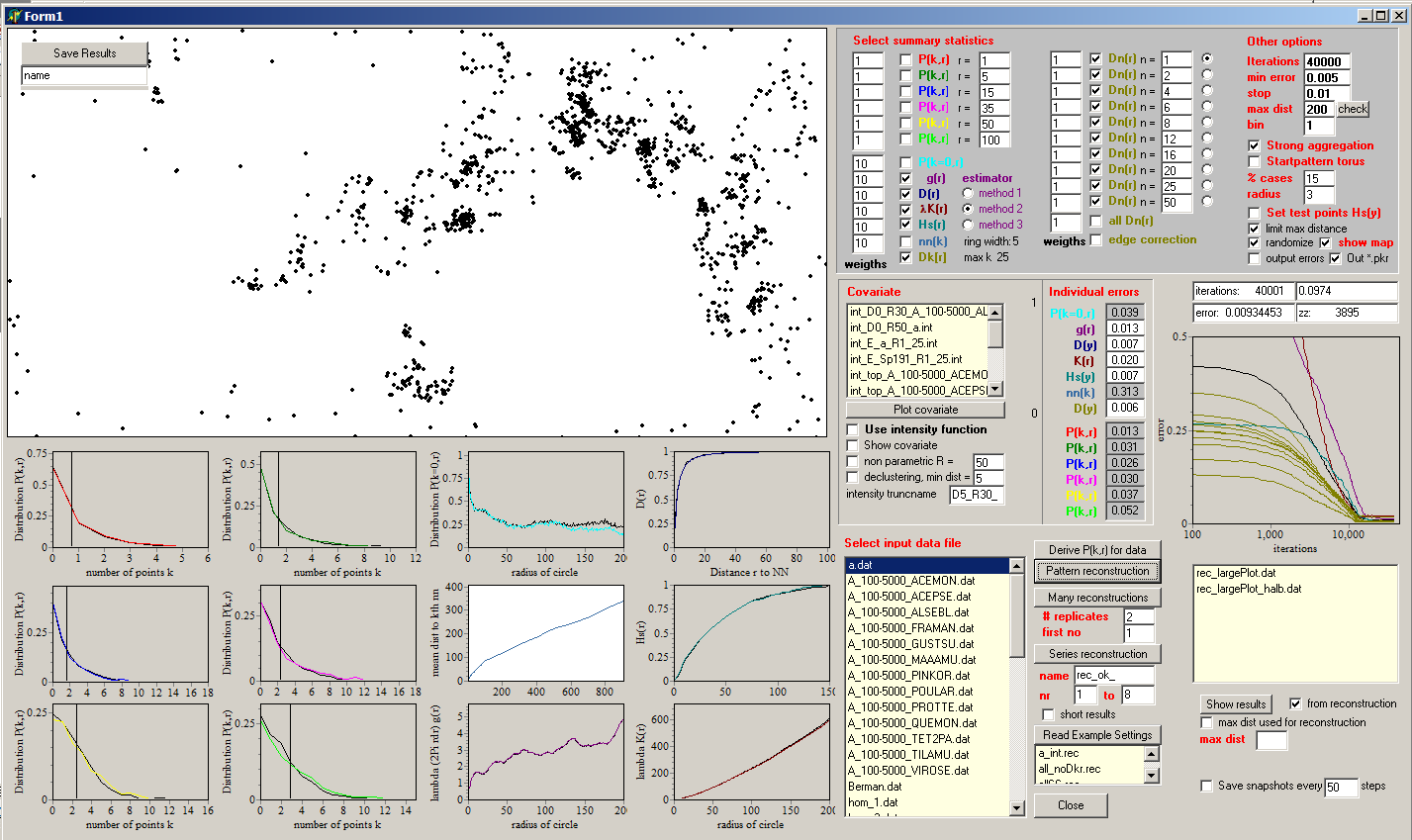## The Pattern reconstruction software

For a number of applications in the study of point patterns, it is useful to generate patterns with predefined properties. For example, null models produce patterns with known properties by holding certain aspects of a pattern fixed, while randomizing other aspects of the data. This is especially difficult in the case of bivariate or multivariate patterns because the null model of independence should conserve the observed univariate structure of the component patterns, but only break their (potential) association. Pattern reconstruction provides a non-parametric solution to this problem because it can produce stochastic replicate patterns, which closely approximate predefined properties of the observed pattern.

Pattern reconstruction uses the information of several summary functions of an observed point pattern to reconstruct point patterns that closely approximate the observed summary functions. This is accomplished by an annealing algorithm that minimizes the difference between the summary functions of the observed and reconstructed pattern.

The procedure starts with a random pattern with the same number of points as the observed pattern. In each simulation step one randomly selected point is tentatively removed and a point with random coordinates is proposed instead. This new point is accepted if the mean difference between the observed and the simulated summary statistics decreases, otherwise it is rejected and another random point is tentatively removed and so on. The reconstruction stops if a predefined number of simulation steps is exceeded or if the mean difference between the observed and the simulated summary statistics is below a predefined threshold value.

The implemented algorithm is an extension of methods presented in Tscheschel and Stoyan (2006) and presented in detail in Wiegand et al. (2013) and Wiegand and Moloney (2014: section 3.4.3). To conduct large parallel reconstruction jobs in an automated way on a computer clusters, the software can also be run in command line mode by using batch files.

For some applications it is desirable to condition on the large-scale intensity function λ(x) of the pattern and to reconstruct only the smaller-scale properties of the pattern. This is implemented by making the selection of the new tentative point dependent on the intensity function λ(x) at location x: the point was rejected with a probability proportional to λ(x). Thus, the reconstruction starts with an initial pattern based on a heterogeneous Poisson process and any further tentative points are selected in accordance with the intensity function λ(x).